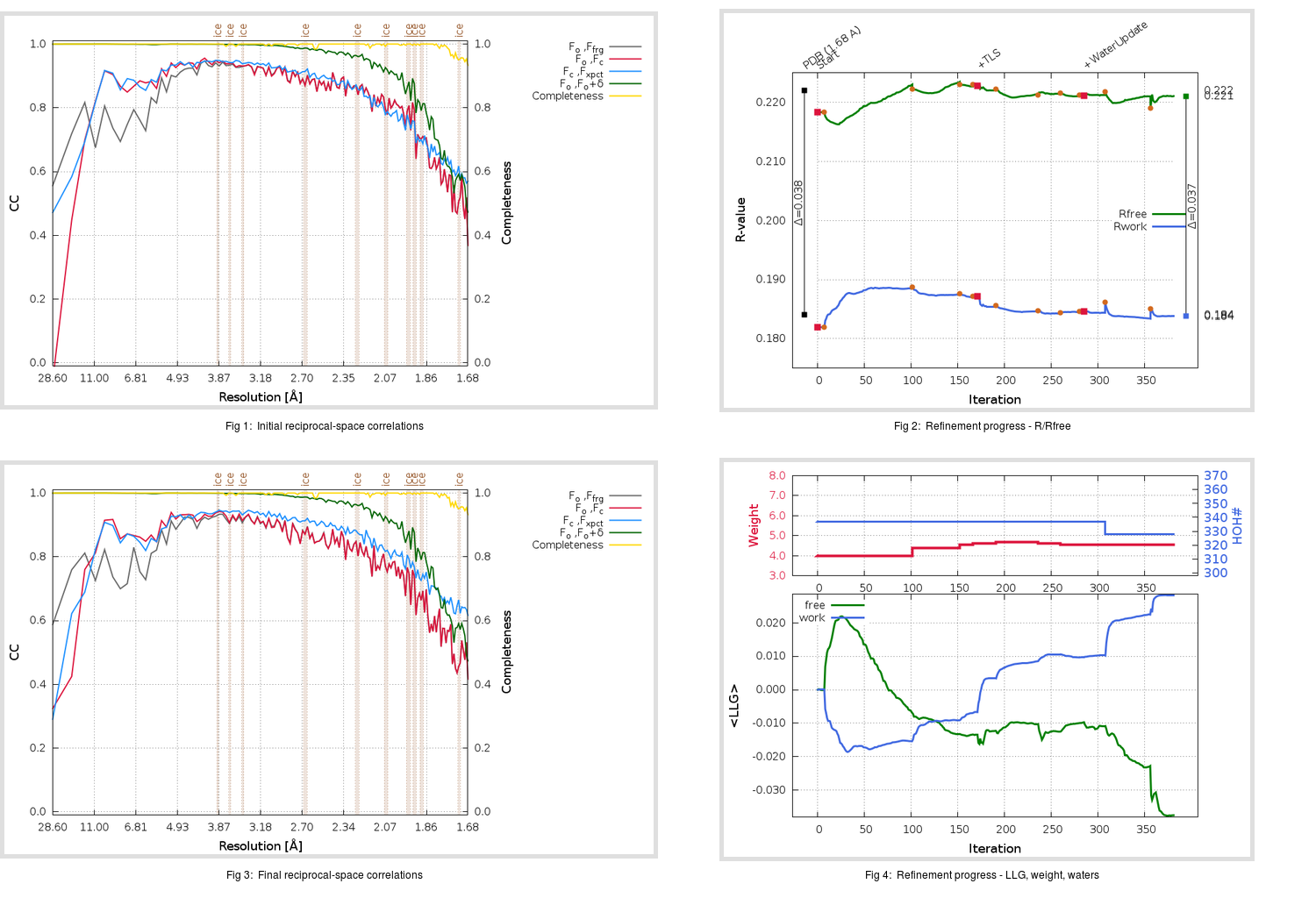Content:

```    Diffraction limits & principal axes of ellipsoid fitted to diffraction cut-off surface:
1.764         0.9982   0.0000  -0.0594       0.994 a* - 0.111 c*
1.752         0.0000   1.0000   0.0000       b*
1.602         0.0594   0.0000   0.9982       0.155 a* + 0.988 c*
```

## Deposited

` `
 Date deposited Date data collection Resolution R, Rfree 20200315 20200304 1.68 0.1820 0.2220

Molprobity (CCP4 7.0 version) summary:

```Ramachandran outliers =   0.33 %
favored =  98.01 %
Rotamer outliers      =   1.89 %
C-beta deviations     =     0
Clashscore            =   7.80
RMS(bonds)            =   0.0134
RMS(angles)           =   1.83
MolProbity score      =   1.64
Resolution            =   1.68
R-work                =   0.1820
R-free                =   0.2220
```

```Number of waters      =   337

<B> (all atoms) =   26.37 ( sd =    9.87 ) for       2740 non-hydrogen atoms
<B>   (protein) =   24.89 ( sd =    9.09 ) for       2370 non-hydrogen atoms
<B>     (water) =   35.95 ( sd =    9.56 ) for        337 non-hydrogen atoms
<B>    (others) =   35.32 ( sd =   11.38 ) for         33 non-hydrogen atoms

B min/max       (all non-hydrogen atoms) =   13.72 /   74.84
B min/max   (protein non-hydrogen atoms) =   13.72 /   74.84
B min/max     (water non-hydrogen atoms) =   15.85 /   60.94
B min/max     (other non-hydrogen atoms) =   23.71 /   51.17
```

## BUSTER (re-)refinement

` `

Molprobity (CCP4 7.0 version) summary:

```Ramachandran outliers =   0.33 %
favored =  98.34 %
Rotamer outliers      =   1.14 %
C-beta deviations     =     0
Clashscore            =   3.37
RMS(bonds)            =   0.0117
RMS(angles)           =   1.56
MolProbity score      =   1.17
Resolution            =   1.68
R-work                =   0.1838
R-free                =   0.2211
```

```Number of waters      =   328

<B> (all atoms) =   27.41 ( sd =    9.16 ) for       2731 non-hydrogen atoms
<B>   (protein) =   25.68 ( sd =    7.54 ) for       2370 non-hydrogen atoms
<B>     (water) =   39.11 ( sd =   10.64 ) for        328 non-hydrogen atoms
<B>    (others) =   34.96 ( sd =   11.80 ) for         33 non-hydrogen atoms

B min/max       (all non-hydrogen atoms) =   10.68 /   85.84
B min/max   (protein non-hydrogen atoms) =   10.68 /   68.20
B min/max     (water non-hydrogen atoms) =   15.79 /   85.84
B min/max     (other non-hydrogen atoms) =   24.65 /   45.29
```

Refinement progression:Results:

` `
 File Remark 5RET_aB_refine.01_03_refine.pdb.gz exact refinement commands are in header 5RET_aB_refine.01_03_refine.mtz.gz including original deposited data and several re-refinement map coefficients 5RET_aB_refine.01_03_BUSTER_model.cif.gz including any non-standard compound restraints 5RET_aB_refine.01_03_BUSTER_refln.cif.gz